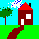HomeUp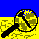Search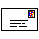Mail
NEW

# Lumped parameters (acoustic -> electrical)

JavaScript calculations can be done below.

The acoustical parameters of the elements can be translated to electrical parameters. I am using information from Calvert , Kinsler (, chapter 8.9 and 10.8) and Colman (pers. comm. ). In the below it is assumed that the acoustic circuit is in size much smaller compared to the acoustical wavelength and that it is the air.

I am wondering how well the below theoretical model works for attenuation/resistance, reading this:
The attenuation of sound in air due to viscous, thermal and rotational loss mechanisms is simply proportional to f 2. However, losses due to vibrational relaxation of oxygen molecules are generally much greater than those due to the classical processes, and the attenuation of sound varies significantly with temperature, water-vapour content and frequency.
More study is needed to include at least molecules level attenuation and influence of humidity. I hope that within the environment of neolithic buildings these influence are not large (except the humidity perhaps). Help is needed!

## Acoustical inductance of a pipe

 L' = ρL/(πa2) [H'] Kinsler , formula (10.8.1) and (10.9.5)

with:
ρ = air density: 1.293 [kg/m3] (0°C, 1 atm)
L = effective length of pipe [m]
a = radius of pipe [m2]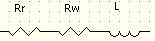## Acoustical resistance of a pipe

### Thermal and viscous resistance due wall losses in pipe

#### Non capillary pipe

 Rw' = (1.46*L/(πa3))√(4πρηf) [Ohm'] Kinsler , formula (10.8.10) and (10.9.5)

with:
η = dynamic viscosity of air, 1.71 x 10-5 [kg/m-sec] (0°C, 1 atm)
f = frequency [Hz]

#### Capillary pipe (Reynolds number is less than 2000)

 Rwc' = 1.46*8ηL/(πa4) [Ohm'] Oban , factor 1.46: Kinsler , formula (8.9.19)

 Rr' = 2πρf2/c (flanged) [Ohm'] Kinsler , formula (10.8.9) and (10.9.5) Rr' = πρf2/c (unflanged) [Ohm'] Kinsler , formula (10.8.9) and (10.9.5)

## Acoustical capacitance of rigid container/cavity

 C' = V/(ρc2) [F'] Kinsler , formula (10.8.4) and (10.9.5)

with:
c = sound speed in air: 331.5 [m/sec] (0°C, 1 atm)
V = volume of container [m3]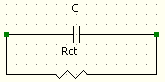### Thermal resistance in container

 Rct' = ρc2/(0.46*√(πηf/ρ)*A) [Ohm'] Coltman, pers. comm 

with:
A = area of cylinder with radius a and length 2*a, where a is such that the cylinder has volume V

## Helmholtz resonator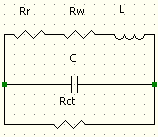### Frequency

 fhelm = 1/(2π√(L'C')) [Hz] Calvert 

### Quality factor

 Qhelm = 2πfhelmL'*( 1/(Rw'(fhelm)+ Rr'(fhelm))+1/Rct') [-]

## Calculations

#### Browser must have JavaScript enabled As example a Florence flask is used.

Temperature:       [ºC]
Air pressure:      [mbar]
Air humidity:      [%] (not implemented yet)

Effective length:  [m]
Frequency:         [Hz]
Flanged:           (flange must be bigger then wavelength)
Volume container:  [m3]

Air density:       [kg/m3]
Air speed:         [m/sec]
Air viscosity:     [kg/m-sec]
L':                E [H']
Rw':               [Ohm']
Rwc':              [Ohm']
Rr':               [Ohm']
Rt':               [Ohm'] {max(Rw',Rwc')+Rr'}
C':                E [F']
Rct':              E [Ohm']

fhelm:              [Hz]
Qhelm:
[-]
Rthelm':            [Ohm'] {1/(1/(Rw(fhelm)'+Rr(fhelm)')+1/Rct(fhelm)')}HomeUpSearchMail